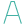#DOLLAR (PQL - xl)

This function converts a number to text using currency format, with the decimals rounded to the specified place

• Library: PQL \ Spreadsheet \ Text
• Compatibility: Any content (regardless of data source) in the Tabulate spreadsheet module

#### Syntax

DOLLAR(number, decimals)

##### Function Arguments
 Name Description Type Optional number Numeric value to convert to text using the currency format Number decimals Number of decimal places to maintain; if negative, the value is rounded to the left of the decimal point; if omitted, the function rounds to two decimal places Number